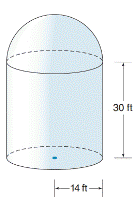Chapter 9.4, Problem 36E### Elementary Geometry for College St...

6th Edition
Daniel C. Alexander + 1 other
ISBN: 9781285195698

#### Solutions

Chapter
Section### Elementary Geometry for College St...

6th Edition
Daniel C. Alexander + 1 other
ISBN: 9781285195698
Textbook Problem
33 views

# An observatory has the shape of a right circular cylinder surmounted by a hemisphere. If the radius of the cylinder is 14 ft and its altitude measures 30 ft, what is the surface area of the observatory? If 1 gallon of paint covers 300   f t   2 , how many gallons are needed to paint the surface if it requires two coats? Use your calculator.To determine

To find:

The surface area and number of gallons of paint required to paint an observatory.

Explanation

Formula Used:

The surface area of a hemisphere S = 2 πr2, where r is the radius of the sphere.

Curved or lateral surface area of a cylinder = 2πrh

Volume of cylinder = πr2h

Calculation:

Consider an observatory that has the shape of a right circular cylinder surmounted by a hemisphere.

The base radius of the cylinder (r) =14 ft and, altitude (h) =30 ft.

Surface area of observatory,

= Lateral surface area of right circular cylinder + Surface area of hemisphere

Surface area of observatory=2πrh+2πr2

Surface area of observatory S=2·3

### Still sussing out bartleby?

Check out a sample textbook solution.

See a sample solution

#### The Solution to Your Study Problems

Bartleby provides explanations to thousands of textbook problems written by our experts, many with advanced degrees!

Get Started

#### Use two disjoint sets to show that 0+2=0.

Mathematical Excursions (MindTap Course List)

#### 28. Find the number A that satisfies for the standard normal z-score.

Mathematical Applications for the Management, Life, and Social Sciences

#### Evaluate the integral, if it exists. x+2x2+4xdx

Single Variable Calculus: Early Transcendentals, Volume I

#### The solution to y = y2 with y(1)=13 is: a) y=1x+2 b) y=lnx+13 c) y=12x216 d) y=12x16

Study Guide for Stewart's Single Variable Calculus: Early Transcendentals, 8th